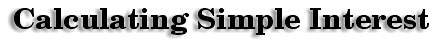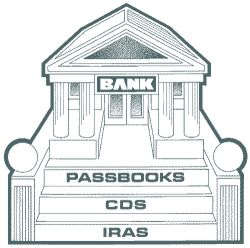Ray just got a full-time job and wants to start saving money so he can raise a family. His brother Bart's advice to Ray was to open a savings account so it can accumulate interest.Beth does not have enough money to pay for college. She will have to take out student loans from the government. As long as she qualifies, there is no limit to how much she can borrow, but she will have to pay back the loans with interest when she graduates.

 Both Beth and Ray’s situations deal with interest. Interest formulas can be quite complicated and difficult to understand. Interest plays a major role in our everyday lives. The simple interest formula is a basic formula that we can use to study interest.Three things are needed to calculate simple interest:Principle = the amount put into the bank or the amount borrowed from the bank Rate = the percent Time = how many years the money is in the savings account at the bank or how many years it will take you to pay back the loan. The formula for calculating interest is very simple: Simple Interest = Principle x Rate x Time (in years) The tricky part about calculating the interest is the time aspect. The time must be in years. If the time is given in months, simply divide your months by 12. This is because there are 12 months in a year.

 Example: Ray put \$1,000 into a savings account. The interest on the account is 3.5%. He wants to put the money away for 18 months. How much will Ray have at the end of that time period? I = p x r x t I = \$1,000 x 3.5% x 18 I = \$1,000 x .035 x 18 (change the percent to a decimal) I = \$1,000 x .035 x 1.5 (divide the number of months by 12) I = \$52.50 Adding the interest back on to the principle, Ray now has \$1,052.50.Beth owes \$38,000 in student loans. The interest rate on her loans is 8.25%. She will be paying these loans off for 20 years. How much will Beth pay altogether? I = px r x t I = \$38,000 x 8.25% x 20 I = \$38,000 x .0825 x 20 (change the percent to a decimal) I = \$62,700 Adding the interest back on to the principle, Beth has to pay \$100,700.

 Luckily for Beth, the president is attempting to pass a law stating the interest on student loan payments cannot exceed 7%. If that is the case, what is the most money that Beth will have to pay?

Calculating Sales Tax and Gratuities (tips)

 mC writer:Kevin Gallagher

Percent